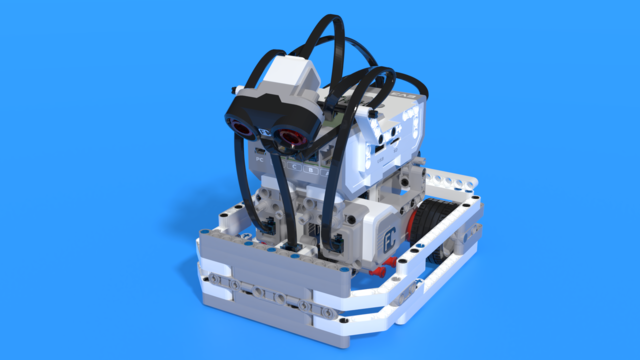# MyBlock for checking if numbers are integers

This block checks if its given number is an integer and returns a logical answer "Yes" if the number is an integer.The way it works is by rounding down the set number and subtracting the result from its original non-rounded value. If the number was an integer, then the rounding did not change it and the result of the subtraction will be 0. If the result is different from 0, then the number was changed during its rounding, which means that it was not an integer.

• #aprqel
• 15 Jan 2020

## Courses and lessons with this Program

This Program is used in the following courses and lessons• 35
• 13:40
• 221• 3
• 8
• 6
• 3d_rotation 1